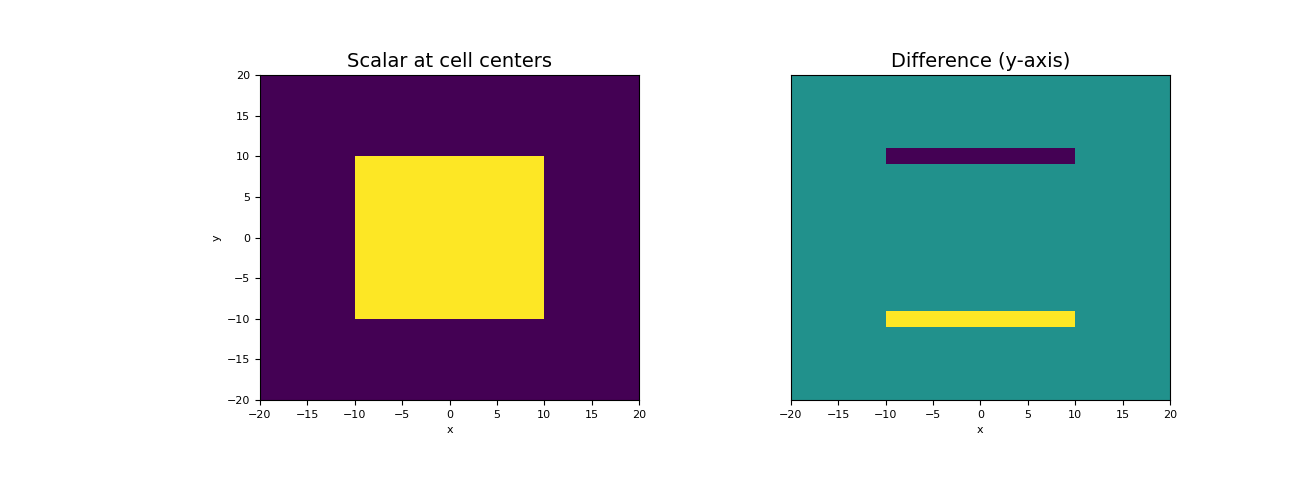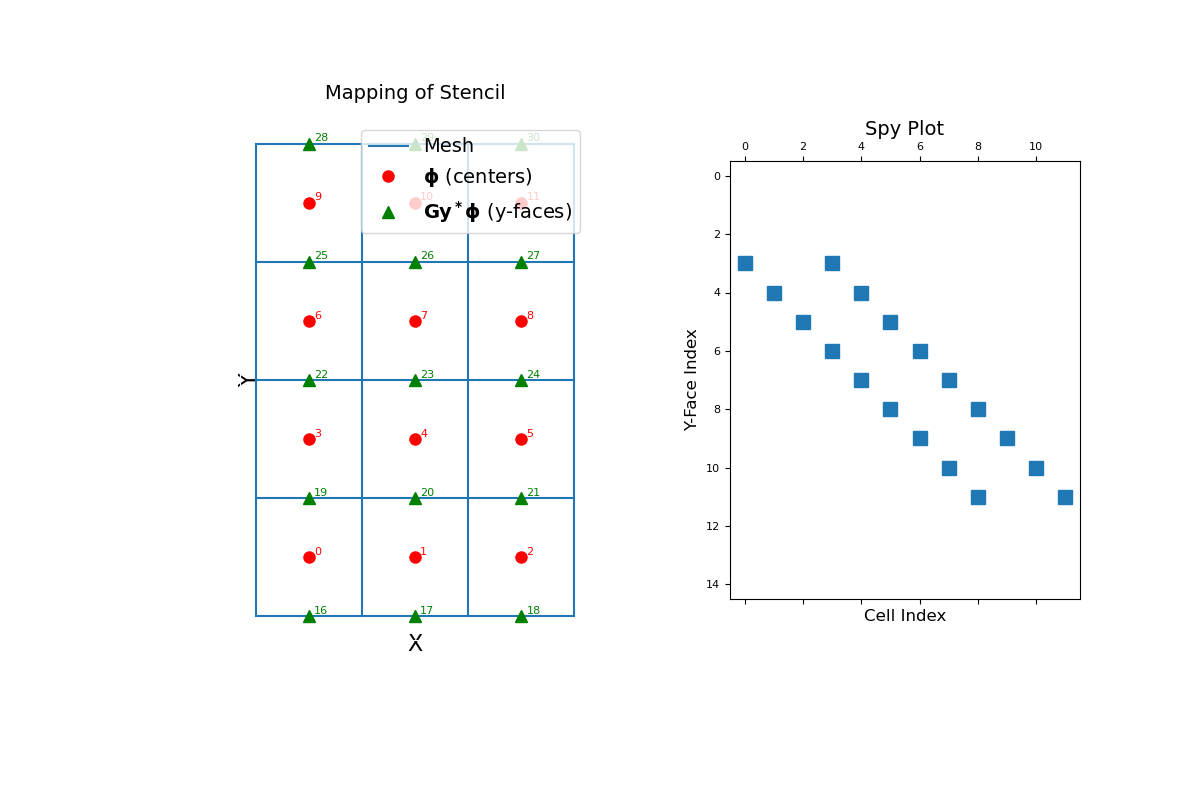Differencing operator along y-direction (cell centers to y-faces)

This property constructs a differencing operator along the x-axis that acts on cell centered quantities; i.e. the stencil for the y-component of the cell gradient. The operator computes the differences between the values at adjacent cell centers along the y-direction, and places the result on the y-faces. The operator is a sparse matrix $$\mathbf{G_y}$$ that can be applied as a matrix-vector product to a cell centered quantity $$\boldsymbol{\phi}$$, i.e.:

diff_phi_y = Gy @ phi

By default, the operator assumes zero-Neumann boundary conditions on the scalar quantity. Before calling stencil_cell_gradient_y however, the user can set a mix of zero Dirichlet and zero Neumann boundary conditions using set_cell_gradient_BC. When stencil_cell_gradient_y is called, the boundary conditions are enforced for the differencing operator.

Returns
(n_faces_y, n_cells) scipy.sparse.csr_matrix

The stencil for the y-component of the cell gradient

Examples

Below, we demonstrate how to set boundary conditions for the y-component cell gradient stencil, construct the operator and apply it to a discrete scalar quantity. The mapping of the operator and its sparsity is also illustrated. Our example is carried out on a 2D mesh but it can be done equivalently for a 3D mesh.

We start by importing the necessary packages and modules.

>>> from discretize import TensorMesh
>>> import numpy as np
>>> import matplotlib.pyplot as plt
>>> import matplotlib as mpl

We then construct a mesh and define a scalar function at cell centers. In this case, the scalar represents some block within a homogeneous medium.

Create a uniform grid

>>> h = np.ones(40)
>>> mesh = TensorMesh([h, h], "CC")

Create a discrete scalar at cell centers

>>> centers = mesh.cell_centers
>>> phi = np.zeros(mesh.nC)
>>> k = (np.abs(mesh.cell_centers[:, 0]) < 10.) & (np.abs(mesh.cell_centers[:, 1]) < 10.)
>>> phi[k] = 1.

Before constructing the operator, we must define the boundary conditions; zero Neumann for our example. Even though we are only computing the difference along y, we define boundary conditions for all boundary faces. Once the operator is created, it is applied as a matrix-vector product.

>>> diff_phi_y = Gy @ phi

Now we plot the original scalar, and the differencing taken along the y-axis.

Expand to show scripting for plot
>>> fig = plt.figure(figsize=(13, 5))
>>> mesh.plot_image(phi, ax=ax1)
>>> ax1.set_title("Scalar at cell centers", fontsize=14)
>>> v = np.r_[np.zeros(mesh.nFx), diff_phi_y]  # Define vector for plotting fun
>>> mesh.plot_image(v, ax=ax2, v_type="Fy")
>>> ax2.set_yticks([])
>>> ax2.set_ylabel("")
>>> ax2.set_title("Difference (y-axis)", fontsize=14)
>>> plt.show()The y-component cell gradient stencil is a sparse differencing matrix that maps from cell centers to y-faces. To demonstrate this, we construct a small 2D mesh. We then show the ordering of the elements and a spy plot.

>>> mesh = TensorMesh([[(1, 3)], [(1, 4)]])
Expand to show scripting for plot
>>> fig = plt.figure(figsize=(12, 8))
>>> mesh.plot_grid(ax=ax1)
>>> ax1.set_title("Mapping of Stencil", fontsize=14, pad=15)
>>> ax1.plot(mesh.cell_centers[:, 0], mesh.cell_centers[:, 1], "ro", markersize=8)
>>> for ii, loc in zip(range(mesh.nC), mesh.cell_centers):
...     ax1.text(loc + 0.05, loc + 0.02, "{0:d}".format(ii), color="r")
>>> ax1.plot(mesh.faces_y[:, 0], mesh.faces_y[:, 1], "g^", markersize=8)
>>> for ii, loc in zip(range(mesh.nFy), mesh.faces_y):
...     ax1.text(loc + 0.05, loc + 0.02, "{0:d}".format(ii + mesh.nFx), color="g")
>>> ax1.set_xticks([])
>>> ax1.set_yticks([])
>>> ax1.spines['bottom'].set_color('white')
>>> ax1.spines['top'].set_color('white')
>>> ax1.spines['left'].set_color('white')
>>> ax1.spines['right'].set_color('white')
>>> ax1.legend(
...     ['Mesh', '$\mathbf{\phi}$ (centers)', '$\mathbf{Gy^* \phi}$ (y-faces)'],
...     loc='upper right', fontsize=14
... )